Trams

Two trams started at the same time from the same place. One tram journey takes 30 minutes and the second 45 minutes to its final stop. How long will trams meet again?

Result

t =  180 min

Solution:Leave us a comment of example and its solution (i.e. if it is still somewhat unclear...):Be the first to comment!To solve this example are needed these knowledge from mathematics:

Do you want to calculate least common multiple two or more numbers?

Next similar examples:

1. Tram lines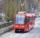Trams of five lines driven at intervals of 5,8,10,12 and 15 minutes. At 12 o'clock come out of the station at the same time. About how many hours again all meet? How many times have earch tram pass for this stop?
2. Runners circle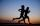Pepa circles the track in 36 seconds. Kamil in 42 seconds. They started together. How many seconds will meet again at the start?
3. Greg and BillGreg is 18 years old. He is 6 less than 4 times Bill's age. How old is Bill?
4. Masons1 mason casts 30.8 meters square in 8 hours. How long casts 4 masons 178 meters square?
5. Grandfather and grandmotherThe old mother is 5 years younger than the old father. Together they are 153 years old. How many years has each of them?
6. Class 9AOn the final certificate have one quarter of the class 9A mark "C" of mathematics, seventh mark "C" from the Czech language and two students failed in chemistry. How many students attend class 9A?
7. Raffle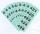In the pool is numbers from 1 to 115. What is the probability that a randomly selected number is not a prime number?
8. TVsProduction of television sets increased from 3,500 units to 4,200 units. Calculate the percentage of production increase.
9. Plum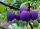On the platter are plum. How many were there if its have to be able to share equally among 8,10 and 12 children?
10. Balls groups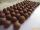Karel pulled the balls out of his pocket and divide it into the groups. He could divide them in four, six or seven, and no ball ever left. How little could be a ball?
11. Lcm simpleFind least common multiple of this two numbers: 140 175.
12. Cents no more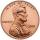Janko bought pencils for 35 cents each. Neither he nor the salesperson had small coins just a whole € 1 coin. At least how many pencils had to buy to pay for the whole euros?
13. EquationSolve the equation: 1/2-2/8 = 1/10; Write the result as a decimal number.
14. InequationSolve the inequation: 5k - (7k - 1)≤ 2/5 . (5-k)-2
15. Unknown numberIdentify unknown number which 1/5 is 40 greater than one tenth of that number.
16. Down syndromeDown syndrome is one of the serious diseases caused by a gene mutation. Down syndrome occurs in approximately every 550-born child. Express the incidence of Down's syndrome in newborns at per mille.
17. Conference148 is the total number of employees. The conference was attended by 22 employees. How much is it in percent?